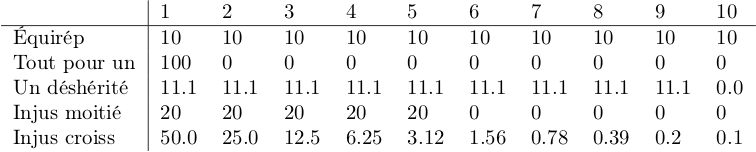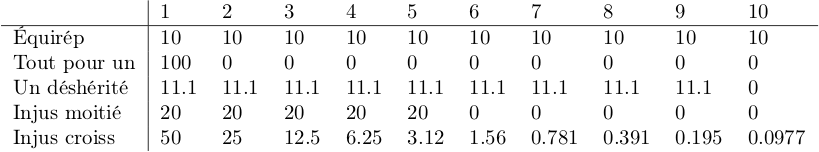# Erasing some of the decimals in a list

This is a nice table to the reserve of the to long decimals

A=[[10,10,10,10,10,10,10,10,10,10],[100,0,0,0,0,0,0,0,0,0],[11.1,11.1,11.1,11.1,11.1,11.1,11.1,11.1,11.1,0]
,[20,20,20,20,20,0,0,0,0,0],[50,25,12.5,6.25,3.125,1.56,0.78,0.39,0.19,0.09]]
t=table(A,header_row=["$1$","$2$","$3$","$4$","$5$","$6$","$7$","$8$","$9$","$10$"],header_column=["","Équi-Rep","Tout pour un","Un déshérité","Injuste pour 1/2","$5$","Injuste croissante"])
show(t)


I have tried to construct a function f(x)=round(x,2) and then to use A.map_apply(f) but this doesnot works since f(x)=round(x,2) generates an error. How shouild I do ?

edit retag close merge delete

Sort by » oldest newest most voted

Here is a solution taking advantage of the following:

• elements of RDF are represented with no trailing decimal zeros
• matrices over RDF have a round method that can round to n decimal digits

There might be simpler ways, but hopefully this helps.

Define A as a list of lists:

sage: A = [ * 10,  +  * 9, [100/9] * 9 + ]
sage: A.extend([ * 5 +  * 5, [50/2**n for n in range(10)]])


Make a deep copy, so we can change it without changing A:

sage: D = deepcopy(A)


Round the rows with non-integer values:

sage: D = list(matrix(RDF, A).round(1))
sage: D = list(matrix(RDF, A).round(2))


sage: hr = [f"${n}$" for n in (1 .. 10)]
sage: hc = ["", "Équirép", "Tout pour un", "Un déshérité"]
sage: hc += ["Injus moitié", "Injus croiss"]


Build the table:

sage: t = table(D, header_row=hr, header_column=hc)


The table in text mode:

sage: t
| $1$    $2$    $3$    $4$    $5$    $6$    $7$    $8$    $9$    $10$
+--------------+------+------+------+------+------+------+------+------+------+------+
Équirép      | 10     10     10     10     10     10     10     10     10     10
Tout pour un | 100    0      0      0      0      0      0      0      0      0
Un déshérité | 11.1   11.1   11.1   11.1   11.1   11.1   11.1   11.1   11.1   0.0
Injus moitié | 20     20     20     20     20     0      0      0      0      0
Injus croiss | 50.0   25.0   12.5   6.25   3.12   1.56   0.78   0.39   0.2    0.1


The latexed table:

sage: view(t)Simpler solution, inspired by the answer given by @dsejas.

Here A, hr, hc are as above.

Round only non-integer entries; adapt rounding precision to get 3 significant digits:

sage: f = lambda a: a if a in ZZ else round(a, 2 - floor(log(a, 10)))
sage: D = [[f(a) for a in r] for r in A]


Build the table:

sage: t = table(D, header_row=hr, header_column=hc)


The table in text mode:

sage: t
| $1$    $2$    $3$    $4$    $5$    $6$    $7$     $8$     $9$     $10$
+--------------+------+------+------+------+------+------+-------+-------+-------+--------+
Équirép      | 10     10     10     10     10     10     10      10      10      10
Tout pour un | 100    0      0      0      0      0      0       0       0       0
Un déshérité | 11.1   11.1   11.1   11.1   11.1   11.1   11.1    11.1    11.1    0
Injus moitié | 20     20     20     20     20     0      0       0       0       0
Injus croiss | 50     25     12.5   6.25   3.12   1.56   0.781   0.391   0.195   0.0977


The latexed table:

sage: view(t)more

Hello, @Cyrille! I believe that @slelievre has already given you a perfect solution for your problem, so i am just going to complement it with a few comments and alternatives for you, or any reader looking for the way to work with maps. However, I highly recommend using @slelievre's answer, which is way more efficient and elegant.

You cannot define f(x) = round(x, 2), because this is a symbolic definition, but round() is not a symbolic function. See the answer to this question for more details (I also made a similar mistake.) Remember that syntax like f(x) = x^2 is actually syntactic sugar defined by Sage for comfort. Instead, when you can't use that type of definitions, you should use a Python function definition:

def f(x):
return round(x, 2)


Now, a similar approach to yours would be to use the map() function; this acts on the elements of a list. In your particular case, A is a list of lists, so you should define a function that takes a list (a row of your table) and returns a list with its elements rounded off (a new row of your table):

def f(row): # row is a row of your table "A" (automatically chosen by "map()")
result = [] # this will be the new rounded off row
for x in row:
result.append(round(x, 2)) # this applies the "round()" function to every element of "row" and appends it to the new row
return result


Now, you can apply this to your table A with this code:

A=[[10,10,10,10,10,10,10,10,10,10],[100,0,0,0,0,0,0,0,0,0],
[11.1,11.1,11.1,11.1,11.1,11.1,11.1,11.1,11.1,0],
[20,20,20,20,20,0,0,0,0,0],[50,25,12.5,6.25,3.125,1.56,0.78,0.39,0.19,0.09]]
temp = map(f, A)


Now temp is a Python map that will round your table elements. In order to trigger the process and obtain a new table (list of lists), you must write list(A), like in the following code:

t=table(list(temp),header_row="$1$","$2$","$3$","$4$","$5$","$6$","$7$","$8$","$9$","$10$"],
header_column["","ÉquiRep","Tout pour un","Un déshérité","Injuste pour 1/2","$5$",
"Injuste croissante"])
show(t)


Following @slelievre's answer, you can also use the following function:

 def f(row):
result = []
for x in row:
result.append(RDF(x))
return result


Once again, this syntax is necessary, since RDF() is not a symbolic function.

I hope this helps!

more

Note: you probably meant list(temp) rather than list(A).

Note: with A as in the question, a maybe simpler way to use round would be:

sage: f = lambda x: round(x, 2)
sage: D = [[f(x) for x in row] for row in A]


or if one likes using map:

sage: f = lambda x: round(x, 2)
sage: ff = lambda row: list(map(f, row))
sage: D = list(map(ff, A))


and then

sage: t = table(D, ...)


Opps, yes, I meant list(temp). I am correcting it now.

Also, thank you for the suggested code simplifications.

No problem. I like using comprehensions instead of initialising an empty list and appending in a for loop.

I find it more concise, elegant and expressive. It may be faster too.

Same for dictionaries, see Stack Overflow answer 64949287.